10.7 Parametric equations: graphs  (Page 4/4)

 Page 4 / 4

Graph on the domain $\text{\hspace{0.17em}}\left[-\pi ,0\right],\text{\hspace{0.17em}}$ where $\text{\hspace{0.17em}}a=5\text{\hspace{0.17em}}$ and $\text{\hspace{0.17em}}b=4$ , and include the orientation.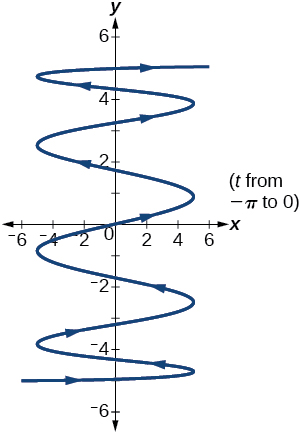If $\text{\hspace{0.17em}}a\text{\hspace{0.17em}}$ is 1 more than $\text{\hspace{0.17em}}b,\text{\hspace{0.17em}}$ describe the effect the values of $\text{\hspace{0.17em}}a\text{\hspace{0.17em}}$ and $\text{\hspace{0.17em}}b\text{\hspace{0.17em}}$ have on the graph of the parametric equations.

Describe the graph if $\text{\hspace{0.17em}}a=100\text{\hspace{0.17em}}$ and $\text{\hspace{0.17em}}b=99.$

There will be 100 back-and-forth motions.

What happens if $\text{\hspace{0.17em}}b\text{\hspace{0.17em}}$ is 1 more than $\text{\hspace{0.17em}}a?\text{\hspace{0.17em}}$ Describe the graph.

If the parametric equations $\text{\hspace{0.17em}}x\left(t\right)={t}^{2}\text{\hspace{0.17em}}$ and $\text{\hspace{0.17em}}y\left(t\right)=6-3t\text{\hspace{0.17em}}$ have the graph of a horizontal parabola opening to the right, what would change the direction of the curve?

Take the opposite of the $\text{\hspace{0.17em}}x\left(t\right)\text{\hspace{0.17em}}$ equation.

For the following exercises, describe the graph of the set of parametric equations.

$x\left(t\right)=-{t}^{2}\text{\hspace{0.17em}}$ and $\text{\hspace{0.17em}}y\left(t\right)\text{\hspace{0.17em}}$ is linear

$y\left(t\right)={t}^{2}\text{\hspace{0.17em}}$ and $\text{\hspace{0.17em}}x\left(t\right)\text{\hspace{0.17em}}$ is linear

The parabola opens up.

$y\left(t\right)=-{t}^{2}\text{\hspace{0.17em}}$ and $\text{\hspace{0.17em}}x\left(t\right)\text{\hspace{0.17em}}$ is linear

Write the parametric equations of a circle with center $\text{\hspace{0.17em}}\left(0,0\right),$ radius 5, and a counterclockwise orientation.

$\left\{\begin{array}{l}x\left(t\right)=5\mathrm{cos}t\\ y\left(t\right)=5\mathrm{sin}t\end{array}$

Write the parametric equations of an ellipse with center $\text{\hspace{0.17em}}\left(0,0\right),$ major axis of length 10, minor axis of length 6, and a counterclockwise orientation.

For the following exercises, use a graphing utility to graph on the window $\text{\hspace{0.17em}}\left[-3,3\right]\text{\hspace{0.17em}}$ by $\text{\hspace{0.17em}}\left[-3,3\right]\text{\hspace{0.17em}}$ on the domain $\text{\hspace{0.17em}}\left[0,2\pi \right)\text{\hspace{0.17em}}$ for the following values of $\text{\hspace{0.17em}}a\text{\hspace{0.17em}}$ and $\text{\hspace{0.17em}}b$ , and include the orientation.

$\left\{\begin{array}{l}x\left(t\right)=\mathrm{sin}\left(at\right)\\ y\left(t\right)=\mathrm{sin}\left(bt\right)\end{array}$

$a=1,b=2$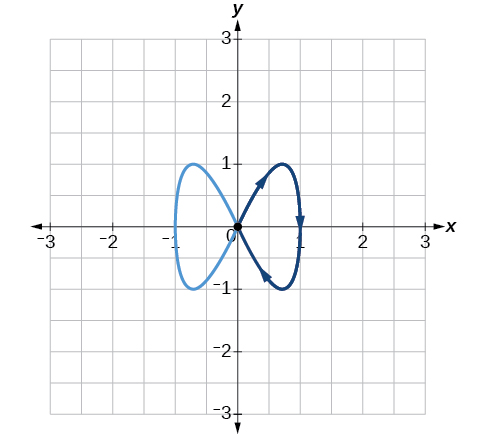$a=2,b=1$

$a=3,b=3$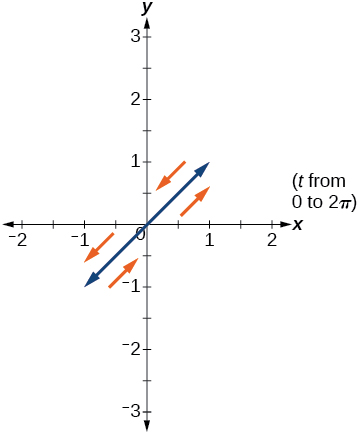$a=5,b=5$

$a=2,b=5$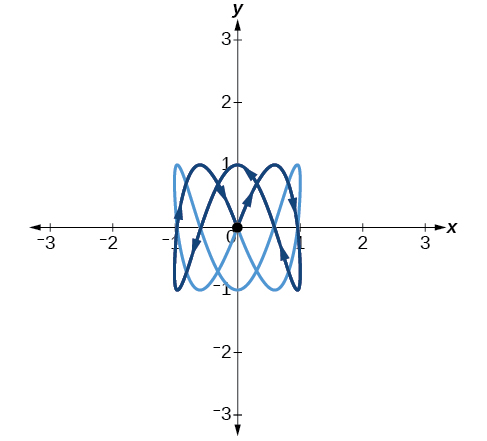$a=5,b=2$

Technology

For the following exercises, look at the graphs that were created by parametric equations of the form $\text{\hspace{0.17em}}\left\{\begin{array}{l}x\left(t\right)=a\text{cos}\left(bt\right)\hfill \\ y\left(t\right)=c\text{sin}\left(dt\right)\hfill \end{array}.\text{\hspace{0.17em}}$ Use the parametric mode on the graphing calculator to find the values of $a,b,c,$ and $d$ to achieve each graph.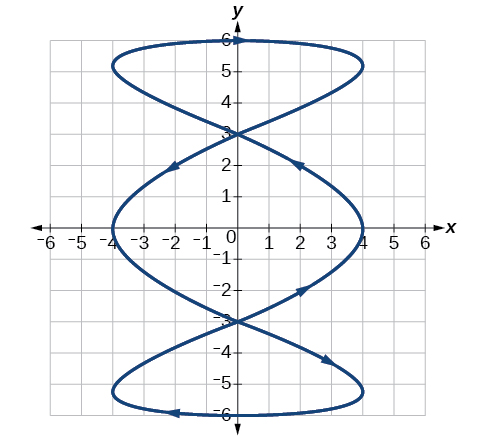$a=4,\text{\hspace{0.17em}}b=3,\text{\hspace{0.17em}}c=6,\text{\hspace{0.17em}}d=1$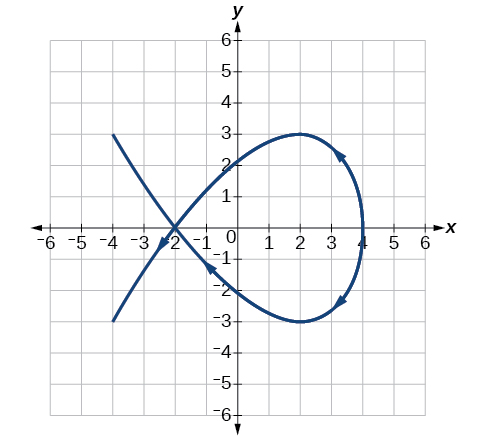$a=4,\text{\hspace{0.17em}}b=2,\text{\hspace{0.17em}}c=3,\text{\hspace{0.17em}}d=3$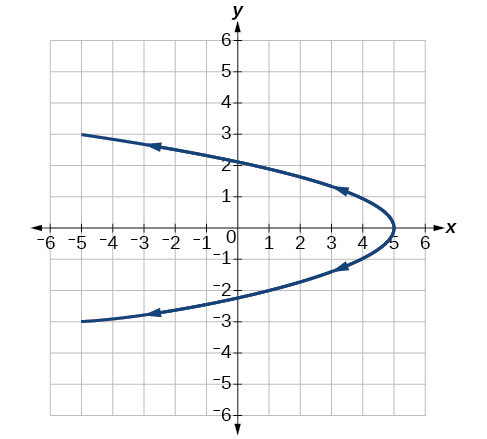For the following exercises, use a graphing utility to graph the given parametric equations.

1. $\left\{\begin{array}{l}x\left(t\right)=\mathrm{cos}t-1\\ y\left(t\right)=\mathrm{sin}t+t\end{array}$
2. $\left\{\begin{array}{l}x\left(t\right)=\mathrm{cos}t+t\\ y\left(t\right)=\mathrm{sin}t-1\end{array}$
3. $\left\{\begin{array}{l}x\left(t\right)=t-\mathrm{sin}t\\ y\left(t\right)=\mathrm{cos}t-1\end{array}$

Graph all three sets of parametric equations on the domain $\text{\hspace{0.17em}}\left[0,\text{\hspace{0.17em}}2\pi \right].$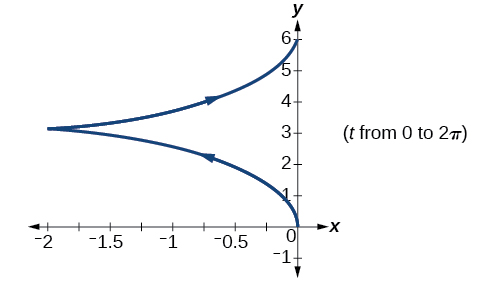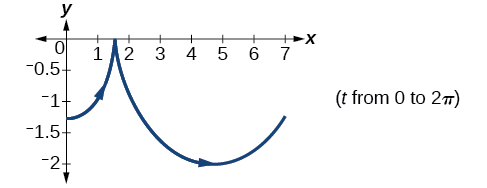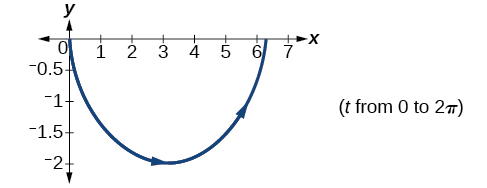Graph all three sets of parametric equations on the domain $\text{\hspace{0.17em}}\left[0,4\pi \right].$

Graph all three sets of parametric equations on the domain $\text{\hspace{0.17em}}\left[-4\pi ,6\pi \right].$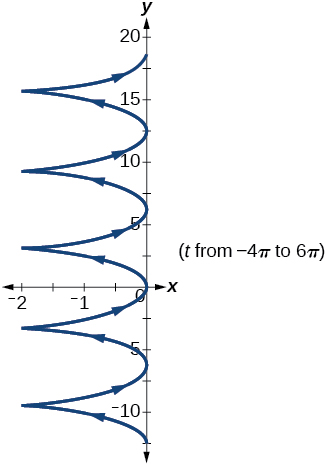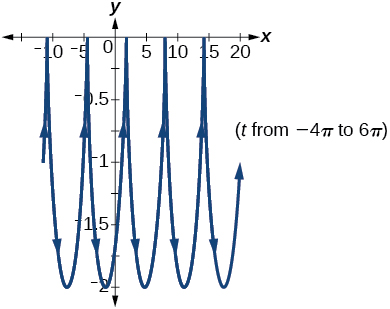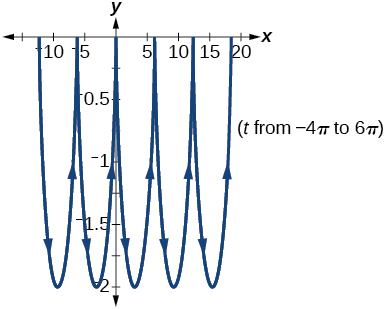The graph of each set of parametric equations appears to “creep” along one of the axes. What controls which axis the graph creeps along?

Explain the effect on the graph of the parametric equation when we switched $\text{\hspace{0.17em}}\mathrm{sin}\text{\hspace{0.17em}}t\text{\hspace{0.17em}}$ and $\text{\hspace{0.17em}}\mathrm{cos}\text{\hspace{0.17em}}t$ .

The $\text{\hspace{0.17em}}y$ -intercept changes.

Explain the effect on the graph of the parametric equation when we changed the domain.

Extensions

An object is thrown in the air with vertical velocity of 20 ft/s and horizontal velocity of 15 ft/s. The object’s height can be described by the equation $\text{\hspace{0.17em}}y\left(t\right)=-16{t}^{2}+20t$ , while the object moves horizontally with constant velocity 15 ft/s. Write parametric equations for the object’s position, and then eliminate time to write height as a function of horizontal position.

$y\left(x\right)=-16{\left(\frac{x}{15}\right)}^{2}+20\left(\frac{x}{15}\right)$

A skateboarder riding on a level surface at a constant speed of 9 ft/s throws a ball in the air, the height of which can be described by the equation $\text{\hspace{0.17em}}y\left(t\right)=-16{t}^{2}+10t+5.\text{}$ Write parametric equations for the ball’s position, and then eliminate time to write height as a function of horizontal position.

For the following exercises, use this scenario: A dart is thrown upward with an initial velocity of 65 ft/s at an angle of elevation of 52°. Consider the position of the dart at any time $\text{\hspace{0.17em}}t.\text{\hspace{0.17em}}$ Neglect air resistance.

Find parametric equations that model the problem situation.

$\left\{\begin{array}{l}x\left(t\right)=64t\mathrm{cos}\left(52°\right)\\ y\left(t\right)=-16{t}^{2}+64t\mathrm{sin}\left(52°\right)\end{array}$

Find all possible values of $\text{\hspace{0.17em}}x\text{\hspace{0.17em}}$ that represent the situation.

When will the dart hit the ground?

approximately 3.2 seconds

Find the maximum height of the dart.

At what time will the dart reach maximum height?

1.6 seconds

For the following exercises, look at the graphs of each of the four parametric equations. Although they look unusual and beautiful, they are so common that they have names, as indicated in each exercise. Use a graphing utility to graph each on the indicated domain.

An epicycloid: $\text{\hspace{0.17em}}\left\{\begin{array}{l}x\left(t\right)=14\mathrm{cos}\text{\hspace{0.17em}}t-\mathrm{cos}\left(14t\right)\hfill \\ y\left(t\right)=14\mathrm{sin}\text{\hspace{0.17em}}t+\mathrm{sin}\left(14t\right)\hfill \end{array}\text{\hspace{0.17em}}$ on the domain $\text{\hspace{0.17em}}\left[0,2\pi \right]$ .

A hypocycloid: $\left\{\begin{array}{l}x\left(t\right)=6\mathrm{sin}\text{\hspace{0.17em}}t+2\mathrm{sin}\left(6t\right)\hfill \\ y\left(t\right)=6\mathrm{cos}\text{\hspace{0.17em}}t-2\mathrm{cos}\left(6t\right)\hfill \end{array}\text{\hspace{0.17em}}$ on the domain $\text{\hspace{0.17em}}\left[0,2\pi \right]$ .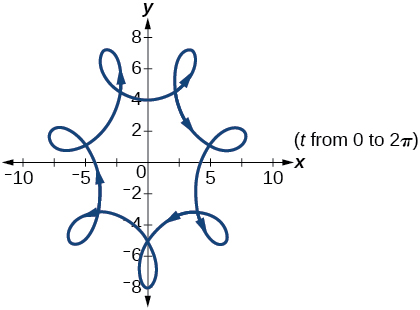A hypotrochoid: $\left\{\begin{array}{l}x\left(t\right)=2\mathrm{sin}\text{\hspace{0.17em}}t+5\mathrm{cos}\left(6t\right)\hfill \\ y\left(t\right)=5\mathrm{cos}\text{\hspace{0.17em}}t-2\mathrm{sin}\left(6t\right)\hfill \end{array}\text{\hspace{0.17em}}$ on the domain $\text{\hspace{0.17em}}\left[0,2\pi \right]$ .

A rose: $\text{\hspace{0.17em}}\left\{\begin{array}{l}x\left(t\right)=5\mathrm{sin}\left(2t\right)\mathrm{sin}t\hfill \\ y\left(t\right)=5\mathrm{sin}\left(2t\right)\mathrm{cos}t\hfill \end{array}\text{\hspace{0.17em}}$ on the domain $\text{\hspace{0.17em}}\left[0,2\pi \right]$ .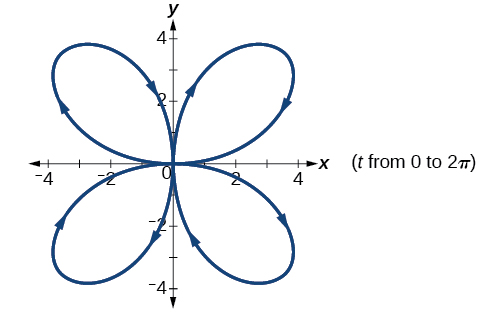A laser rangefinder is locked on a comet approaching Earth. The distance g(x), in kilometers, of the comet after x days, for x in the interval 0 to 30 days, is given by g(x)=250,000csc(π30x). Graph g(x) on the interval [0, 35]. Evaluate g(5)  and interpret the information. What is the minimum distance between the comet and Earth? When does this occur? To which constant in the equation does this correspond? Find and discuss the meaning of any vertical asymptotes.
The sequence is {1,-1,1-1.....} has
how can we solve this problem
Sin(A+B) = sinBcosA+cosBsinA
Prove it
Eseka
Eseka
hi
Joel
June needs 45 gallons of punch. 2 different coolers. Bigger cooler is 5 times as large as smaller cooler. How many gallons in each cooler?
7.5 and 37.5
Nando
find the sum of 28th term of the AP 3+10+17+---------
I think you should say "28 terms" instead of "28th term"
Vedant
the 28th term is 175
Nando
192
Kenneth
if sequence sn is a such that sn>0 for all n and lim sn=0than prove that lim (s1 s2............ sn) ke hole power n =n
write down the polynomial function with root 1/3,2,-3 with solution
if A and B are subspaces of V prove that (A+B)/B=A/(A-B)
write down the value of each of the following in surd form a)cos(-65°) b)sin(-180°)c)tan(225°)d)tan(135°)
Prove that (sinA/1-cosA - 1-cosA/sinA) (cosA/1-sinA - 1-sinA/cosA) = 4
what is the answer to dividing negative index
In a triangle ABC prove that. (b+c)cosA+(c+a)cosB+(a+b)cisC=a+b+c.
give me the waec 2019 questionsByByBy Edward BitonBy